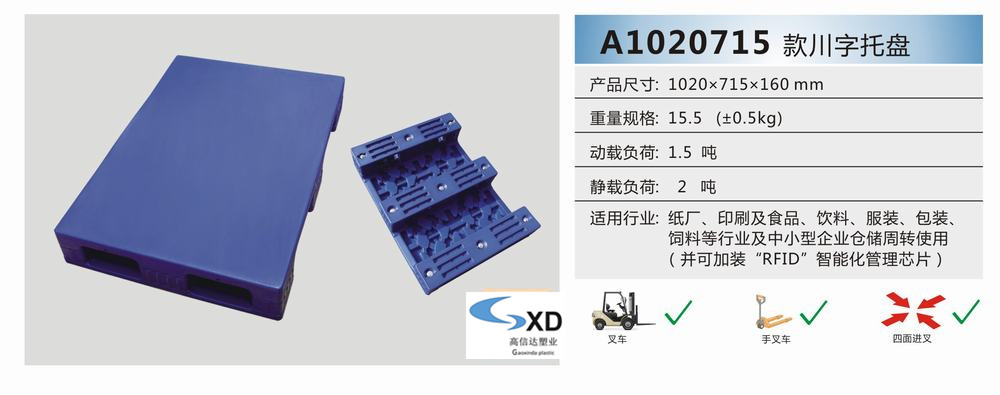QQ：772958196

E-mail：772958196@qq.com1.

1000×800×145   1000×1000×145   1040×760×145

1054×762×172   1100×900×145   1100×1100×145

1140×1140×140   1200×800×145   1200×1000×140（重型）

1200×1000×1400（轻型） 1200×1200×145   1300×1100×140

2.

1020×715×160   1100×1100×160   1200×1000×160A/B

1200×1200×160   1300×1100×160   1400×1200×160

1500×1200×160

3.

1100×1100×160   1200×1100×160   1200×1200×160

1300×1100×160   1400×1200×160   1500×1200×160

1200×1000×150   1200×1200×150   1300×1100×150

1400×1200×150（四面进叉） 1400×1200×150（两面进叉）

1500×1300×150   1500×1500×150   1500×1250×150

1500×1200×150   1500×1400×150   1600×1400×150